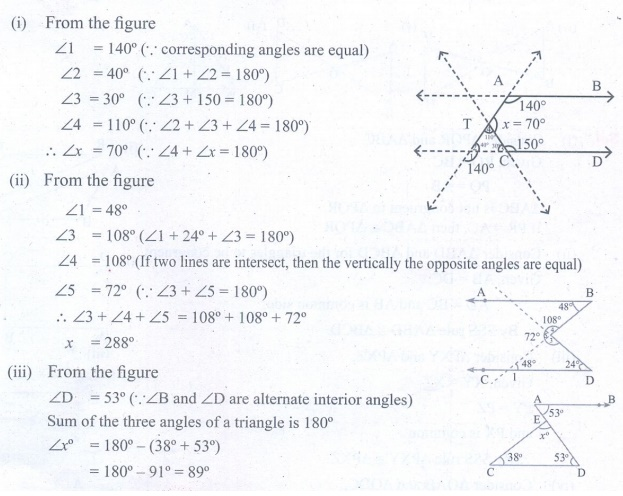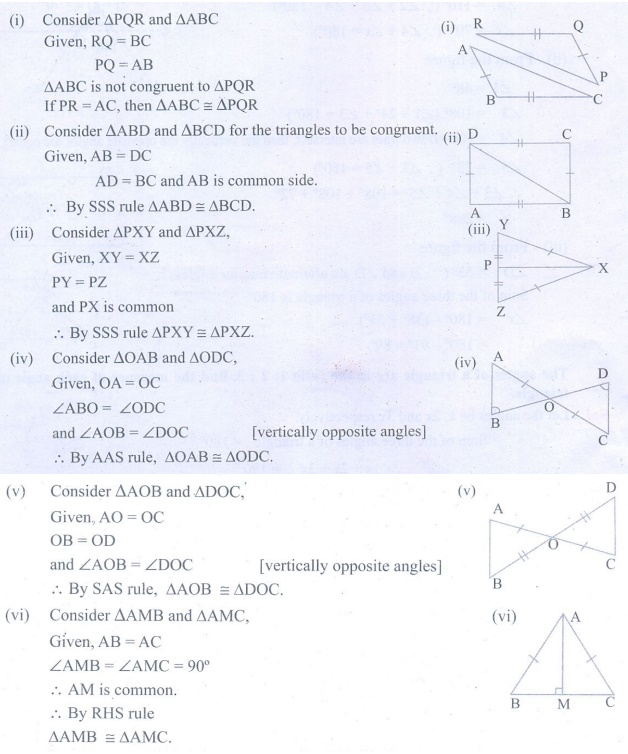Home | | Maths 9th std | Exercise 4.1: Types of Angles, Transversal, Triangles

# Exercise 4.1: Types of Angles, Transversal, Triangles

Maths : Geometry : Book Back, Exercise, Example Numerical Question with Answers, Solution: Exercise 4.1: Types of Angles, Transversal, Triangles

Exercise 4.1

1. In the figure, AB is parallel to CD, find x2. The angles of a triangle are in the ratio 1: 2 : 3, find the measure of each angle of the triangle.3. Consider the given pairs of triangles and say whether each pair is that of congruent triangles. If the triangles are congruent, say ‘how’; if they are not congruent say ‘why’ and also say if a small modification would make them congruent:4. ΔABC and ΔDEF are two triangles in which AB=DF, ACB=70°, ABC=60°; DEF=70° and EDF=60°.

Prove that the triangles are congruent.5. Find all the three angles of the ΔABCTags : Numerical Problems with Answers, Solution | Geometry | Maths , 9th Maths : UNIT 4 : Geometry
Study Material, Lecturing Notes, Assignment, Reference, Wiki description explanation, brief detail
9th Maths : UNIT 4 : Geometry : Exercise 4.1: Types of Angles, Transversal, Triangles | Numerical Problems with Answers, Solution | Geometry | Maths